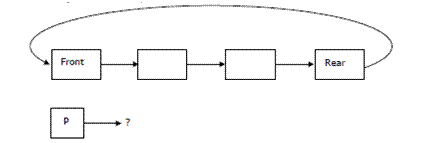Related Articles
Data Structures and Algorithms | Set 2
• Difficulty Level : Easy
• Last Updated : 12 Apr, 2019

Following questions have been asked in GATE CS exam.

1. Consider the function f defined below.

 `struct` `item ``{ ``  ``int` `data; ``  ``struct` `item * next; ``}; `` ` `int` `f(``struct` `item *p) ``{ ``  ``return` `(``          ``(p == NULL) || ``          ``(p->next == NULL) || ``          ``(( P->data <= p->next->data) && f(p->next))``         ``); ``} `

For a given linked list p, the function f returns 1 if and only if (GATE CS 2003)
a) the list is empty or has exactly one element
b) the elements in the list are sorted in non-decreasing order of data value
c) the elements in the list are sorted in non-increasing order of data value
d) not all elements in the list have the same data value.

The function f() works as follows
1) If linked list is empty return 1
2) Else If linked list has only one element return 1
3) Else if node->data is smaller than equal to node->next->data and the same thing holds for rest of the list then return 1
4) Else return 0

2. Consider the label sequences obtained by the following pairs of traversals on a labeled binary tree. Which of these pairs identify a tree uniquely (GATE CS 2004)?
i) preorder and postorder
ii) inorder and postorder
iii) preorder and inorder
iv) level order and postorder

a) (i) only
b) (ii), (iii)
c) (iii) only
d) (iv) only

Please see this post for the explanation.

3. The following numbers are inserted into an empty binary search tree in the given order: 10, 1, 3, 5, 15, 12, 16. What is the height of the binary search tree (the height is the maximum distance of a leaf node from the root)? (GATE CS 2004)
a) 2
b) 3
c) 4
d) 6

Constructed binary search tree will be..

```                    10
/     \
1       15
\      /  \
3    12   16
\
5```

4. A data structure is required for storing a set of integers such that each of the following operations can be done in (log n) time, where n is the number of elements in the set.

```   o    Deletion of the smallest element
o    Insertion of an element if it is not already present in the set
```

Which of the following data structures can be used for this purpose?
(a) A heap can be used but not a balanced binary search tree
(b) A balanced binary search tree can be used but not a heap
(c) Both balanced binary search tree and heap can be used
(d) Neither balanced binary search tree nor heap can be used

A self-balancing balancing binary search tree containing n items allows the lookup, insertion, and removal of an item in O(log n) worst-case time. Since it’s a self-balancing BST, we can easily find out minimum element in O(logn) time which is always the leftmost element (See Find the node with the minimum value in a Binary Search Tree).

Since Heap is a balanced binary tree (or almost complete binary tree), insertion complexity for heap is O(logn). Also, complexity to get minimum in a min heap is O(logn) because removal of root node causes a call to heapify (after removing the first element from the array) to maintain the heap tree property. But a heap cannot be used for the above purpose as the question says – insert an element if it is not already present. For a heap, we cannot find out in O(logn) if an element is present or not. Thanks to the game for providing the correct solution.

5. A circularly linked list is used to represent a Queue. A single variable p is used to access the Queue. To which node should p point such that both the operations enQueue and deQueue can be performed in constant time? (GATE 2004)a) rear node
b) front node
c) not possible with a single pointer
d) node next to the front

 `/* p is pointer to address of rear (double pointer).  This function adds new ``   ``node after rear and updates rear which is *p to point to new node  */``void`  `enQueue(``struct` `node **p, ``struct` `node *new_node)``{``    ``/* Missing code to handle base cases like *p is NULL */``      ` `     ``new_node->next = (*p)->next;``     ``(*p)->next = new_node;``     ``(*p) = new_node ``/* new is now rear */``     ``/* Note that p is again front and  p->next is rear */`` ``}`` ` `/* p is pointer to rear. This function removes the front element and ``    ``returns the new front */``struct` `node *deQueue(``struct` `node *p)``{``    ``/* Missing code to handle base cases like p is NULL,``        ``p->next is NULL,...  etc */`` ` `    ``struct` `node *temp = p->next->next;``    ``p->next = p->next->next;``    ``return` `temp;``    ``/* Note that p is again front and  p->next is rear */``}`